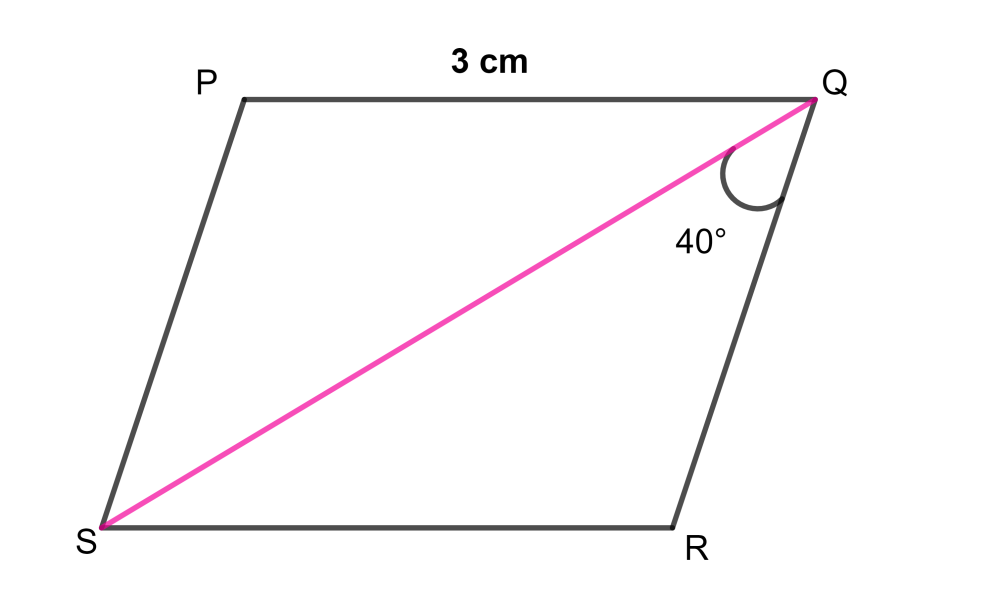QUESTION

# In a Rhombus PQRS, $\angle SQR=40{}^\circ$, and PQ = 3 cm. Find $\angle SPQ\,and\,\angle QSR$ and the perimeter of the rhombus.

Hint: Use the formula “Perimeter of rhombus PQRS = 4 (PQ)” to get the perimeter. As all the sides of rhombus are equal therefore use the concept “In a triangle angles opposite to equal sides are always equal” in $\Delta QSR$ to get the value of $\angle QSR$ and then find the value of $\angle QRS$ by using the property of angles of triangle. Lastly use the property “The opposite angles of a rhombus are equal” to get the values of $\angle SPQ\,$.

To solve the given question we will draw a rhombus with given notations to make the solution easier, therefore,Now we will write the given data as follows,

PQ = 3 cm and $\angle SQR=40{}^\circ$ …………………………………………. (1)

As we know the property of rhombus that all the sides of a Rhombus are equal therefore we can write,

PQ = QR = RS = PS

Comparing above equation with equation (1) we will get,

PQ = QR = RS = PS = 3 cm ………………………………………………… (2)

To find the perimeter of rhombus we should know the formula given below,

Formula:

Perimeter of Rhombus = 4 (Side of Rhombus)

By using above formula we can write the formula of perimeter of rhombus PQRS as follows,

Perimeter of rhombus PQRS = 4 (PQ)

If we put the value of equation (1) in the above equation we will get,

Therefore, Perimeter of Rhombus PQRS = 4 (3)

Therefore, Perimeter of rhombus PQRS = 12 cm ………………………………………. (3)

To find the value of $\angle QSR$ we will take the triangle QSR in consideration, therefore,

Consider$\Delta QSR$,

From equation (2) we can write,

QR = RS

To proceed further in the solution we should know the concept given below,

Concept:

In a triangle angles opposite to equal sides are always equal.

As we know that QR = RS therefore by using the above concept in $\Delta QSR$ we can write,

$\angle QSR=\angle SPQ\,$

If we put the value of equation (1) in the above equation we will get,

$\,\therefore \angle QSR=40{}^\circ \,$ …………………………………………….. (4)

As we know that the sum of all the angles of a triangle is always equal to $180{}^\circ \,$ therefore in $\Delta QSR$ we can write,

$\angle SPQ\,+\angle QSR+\angle QRS=180{}^\circ \,$

If we put the values of equation (1) and equation (4) in the above equation we will get,

$\therefore 40{}^\circ \,+40{}^\circ \,+\angle QRS=180{}^\circ \,$

By simplifying the above equation we will get,

$\therefore 80{}^\circ \,+\angle QRS=180{}^\circ \,$

$\therefore \angle QRS=180{}^\circ -80{}^\circ \,$

$\therefore \angle QRS=100{}^\circ \,$ ……………………………………………………………….. (5)

Now to proceed further in the solution we should know the property of rhombus given below,

Property:

The opposite angles of a rhombus are equal.

By using above concept we can write,

$\angle QRS=\angle SPQ$

If we put the value of equation (5) in the above equation we will get,

$\therefore \angle SPQ=100{}^\circ \,$ …………………………………………………………………… (6)

Now from equation (3), equation (4) and equation (6) we can write our final answer as,

In rhombus PQRS,

Perimeter of rhombus PQRS = 12 cm

$\,\angle QSR=40{}^\circ \,$

$\angle SPQ=100{}^\circ \,$

Note: You can find the value of $\angle SPQ$ by using the concept of alternate angles as the opposite sides of rhombus are parallel to each other. But this procedure is lengthy as we have to find all the angles of $\Delta SPQ$.